# 978-0123869449 Chapter 6 Part 2

Document Type
Homework Help
Book Title
Authors
Michael F. Modest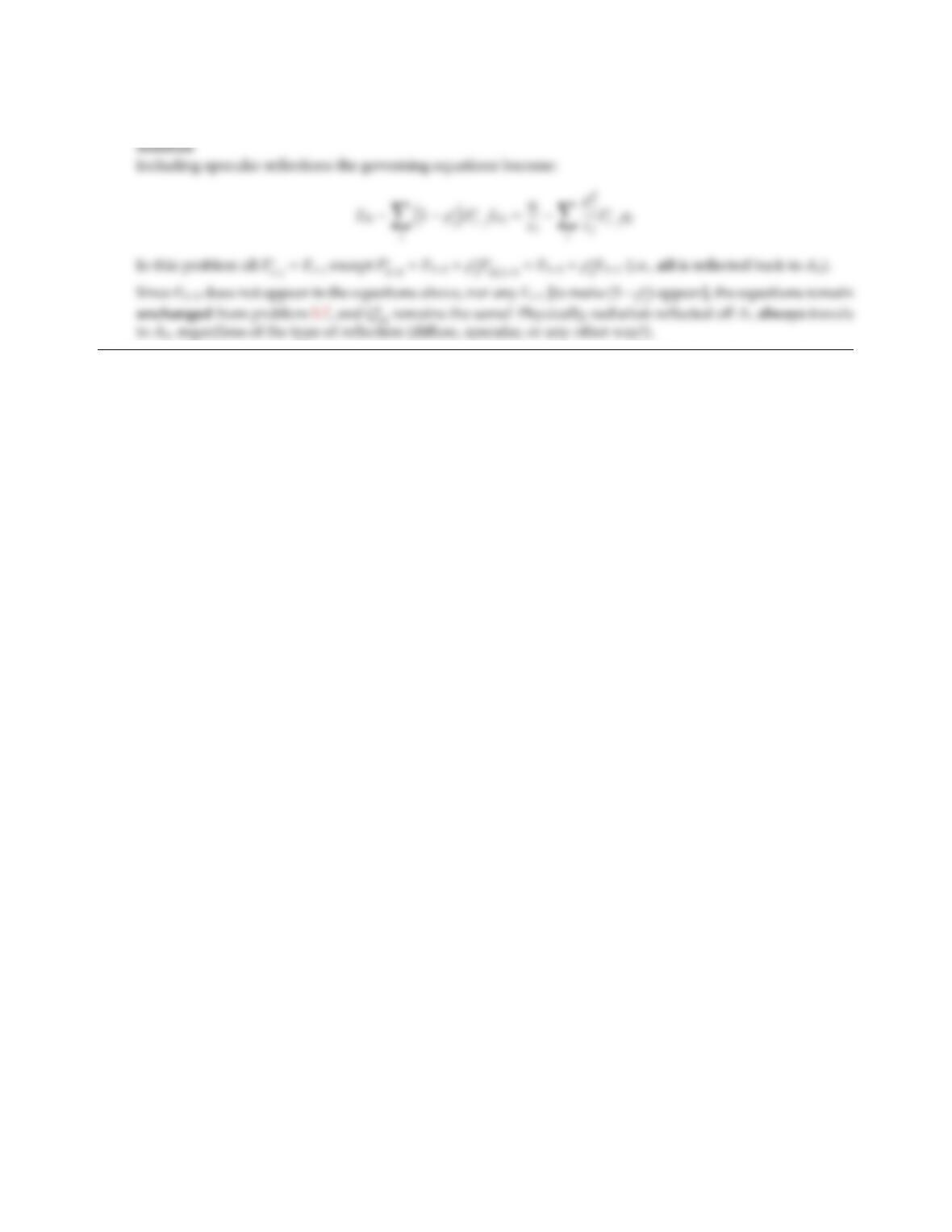6.15 Repeat problem 5.7 for the case that the ﬂat part of the rod (A1)is a purely specular reﬂector.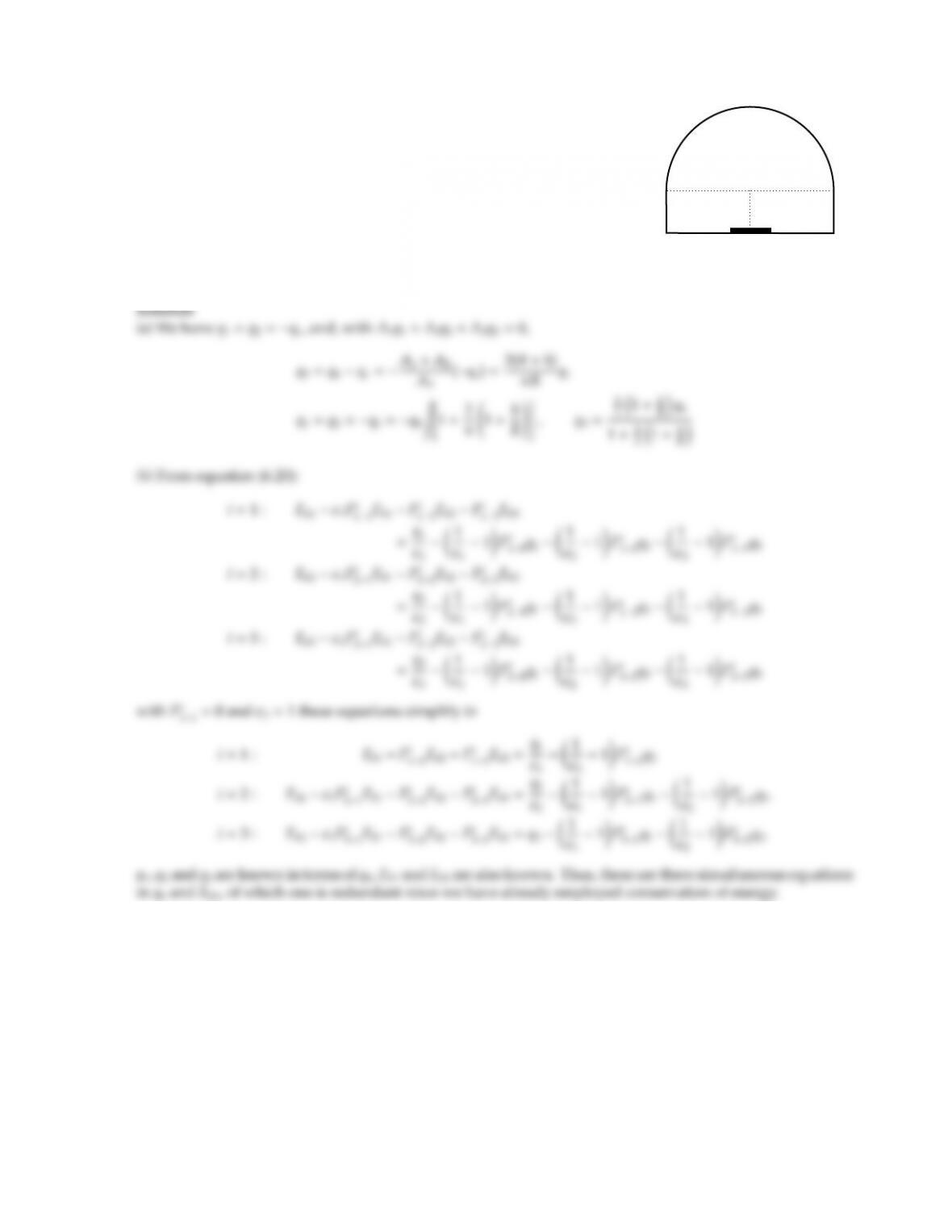CHAPTER 6 189
6.16
A1
A3
A2
A2
A2
R=1m
R
h=0.1m
2w=0.1m
A long furnace may, in a simplified scenario, be considered to consist of
a strip plate (the material to be heated, A1:ǫ1=0.2,T1=500K, specular
reﬂector), unheated refractory brick (ﬂat sides and bottom, A2:ǫ2=0.1,
diuse reﬂector), and a cylindrical dome of heated refractory brick (A3:
ǫ3=1,T3=1000K). Heat release inside the heated brick is qh(W/m2). The
total heat release is radiated into the furnace cavity and is removed by
convection, such that the convective heat loss is uniform everywhere (at qc
W/m2on all three surfaces).
(a) Express the net radiative ﬂuxes on all three surfaces in terms of qh.
(b) Determine the qhnecessary to maintain the indicated temperatures.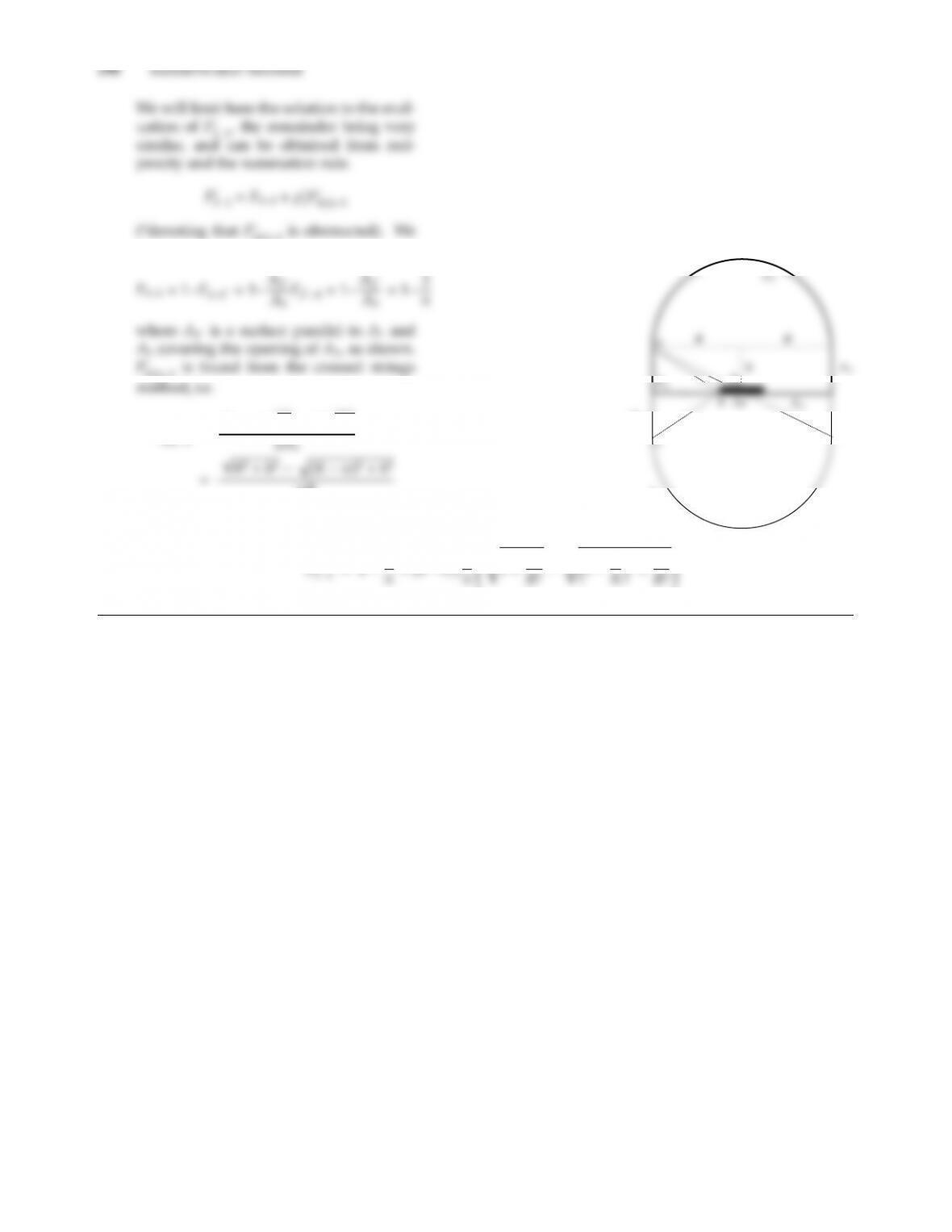3(1)3is obstructed). We
have
3(1)3is found from the crossed strings
F
πR
d
A2(1)
A3(1)
Fs
+h2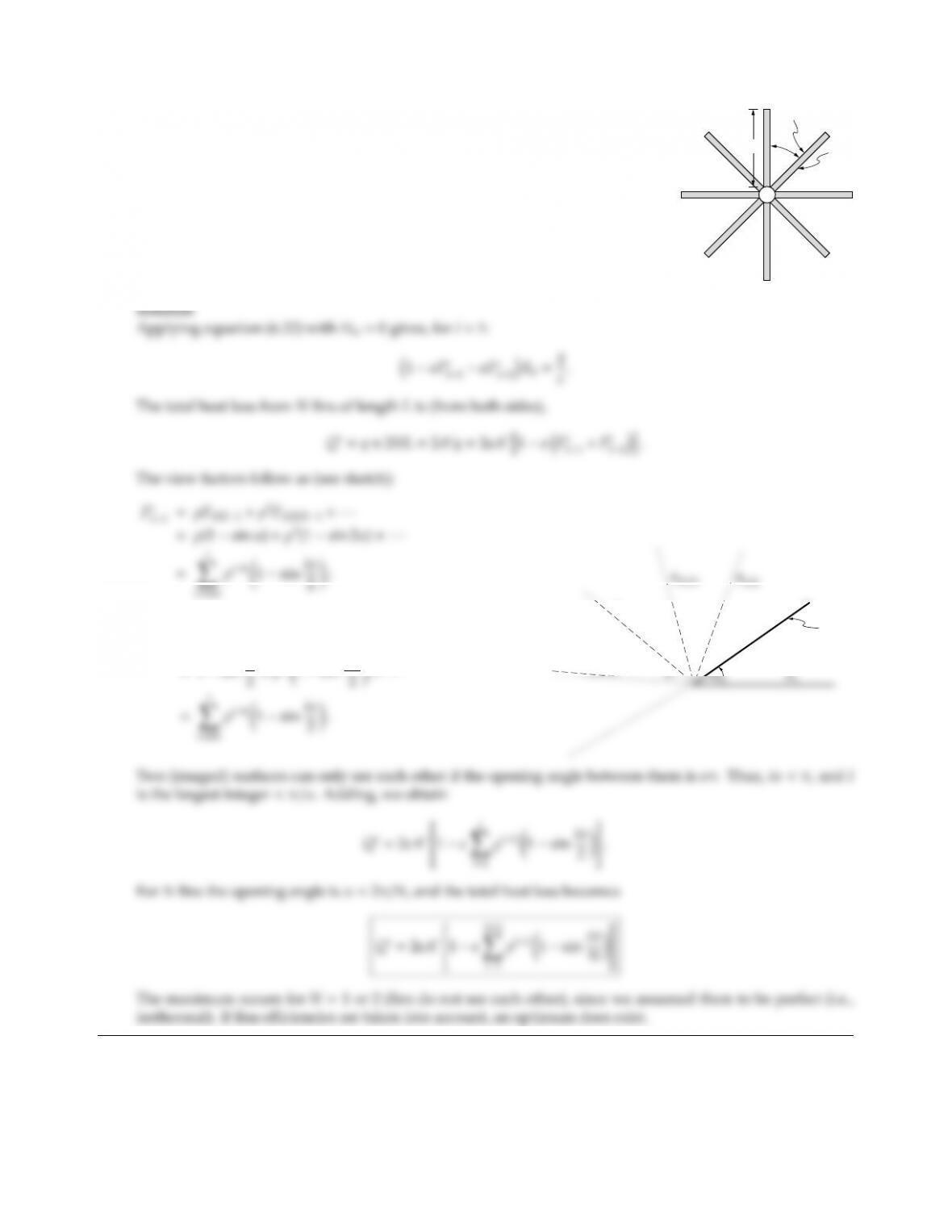CHAPTER 6 191
6.17
α
, T
L
, T
A typical space radiator may have a shape as shown in the adjacent sketch,
i.e., a small tube to which are attached a number of ﬂat plate fins, spaced
at equal angle intervals. Assume that the central tube is negligibly small,
and that a fixed amount of specularly-reﬂecting fin material is available
(ǫ=ρs=0.5), to give (per unit length of tube) a total, one-sided fin area
of A=N×L. Also assume the whole structure to be isothermal. Develop
an expression for the total heat loss from the radiator as a function of the
number of fins (each fin having length L=A/N). Does an optimum exist?
Qualitatively discuss the more realistic case of supplying a fixed amount of
heat to the bases of the fins (rather than assuming isothermal fins).
Fs
12=F12+ρ2F1(21)2+···
=1sin α
A2
α
α
α
α
A1(212)
A2(1212)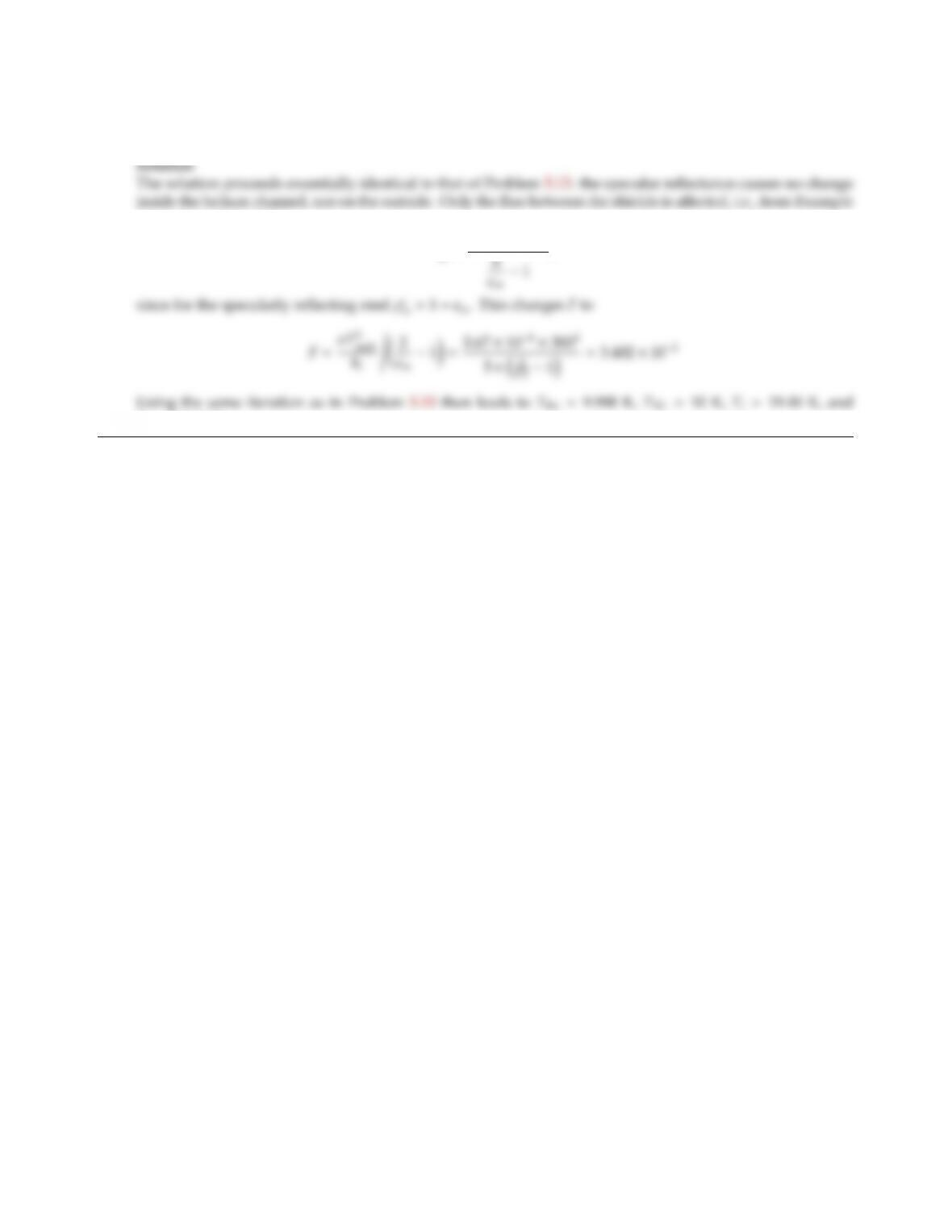6.18 Repeat Problem 5.15 for the case that the stainless steel, while being a gray and diuse emitter, is a purely
specular reﬂector (all four surfaces).
6.4 and equation (6.26),
Q=σAi(T4
oT4
i)
,
To=293.92 K.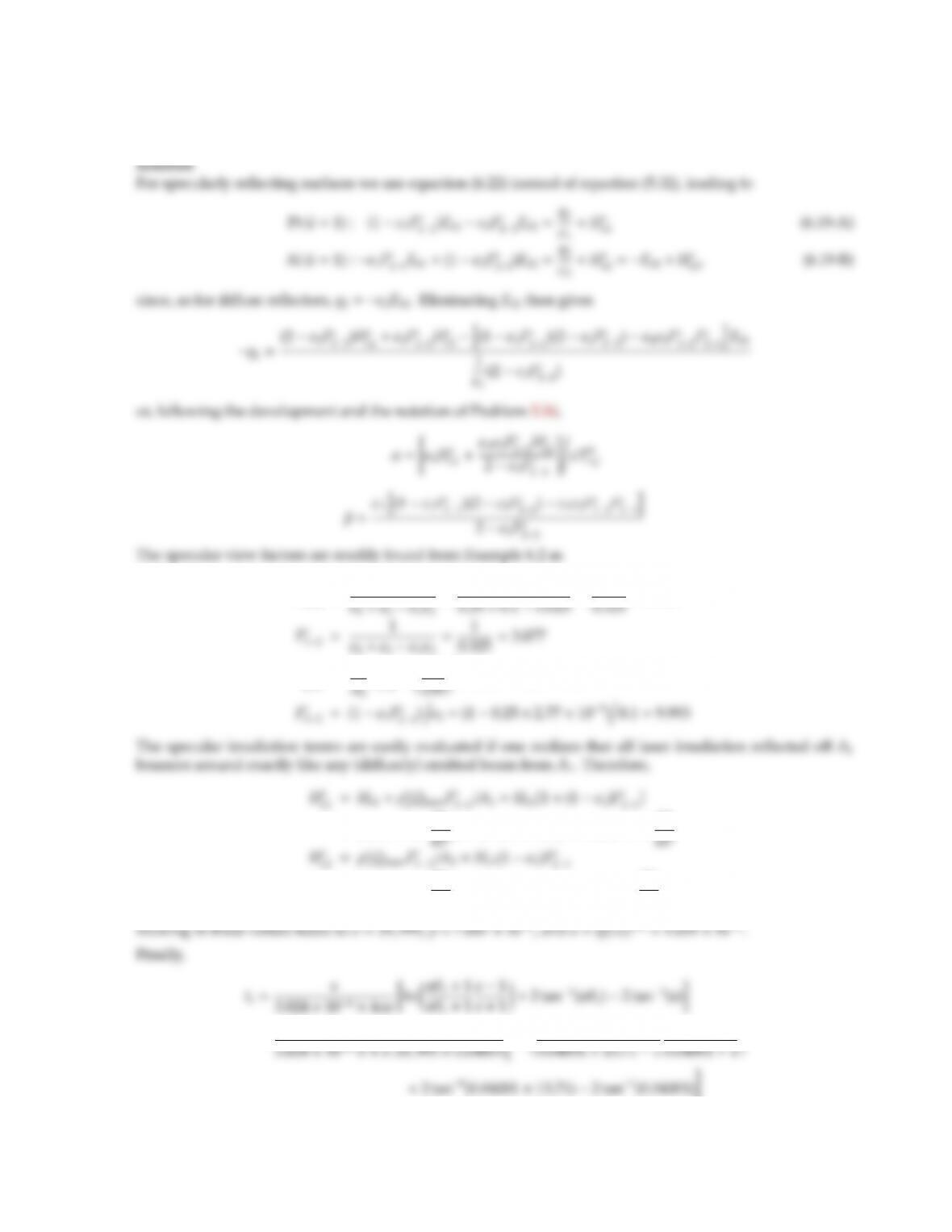CHAPTER 6 193
6.19 Repeat Problem 5.16 for the case that, both, the platinum sphere as well as the aluminum shield, while being
a gray and diuse emitters, are purely specular reﬂectors.
Fs
11=1ǫ2
=0.9
Fs
21=A1
Fs
12=3
×3.077 =2.77 ×103
=3.537 ×106W
=3.537 ×106W
m2×0.75 ×2.77 ×103=7,346 W
m2
=s
0.04001 1te=1.43 s
For specular surfaces the heat-up is considerably faster than for diuse ones: any radiation reﬂected from A1
(diusely or specularly) travels to A2and, if reﬂected specularly, travels back to the platinum sphere (rather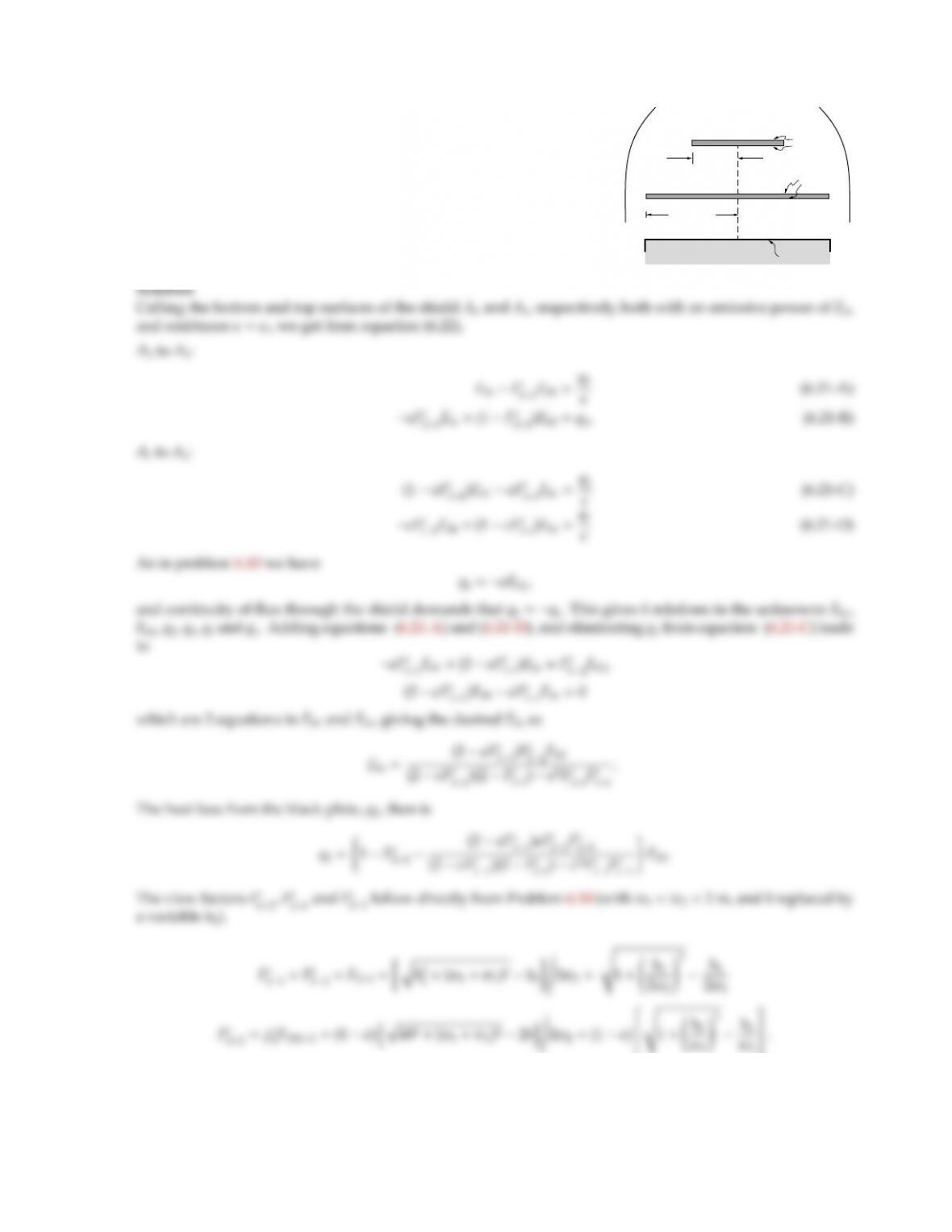CHAPTER 6 195
6.21
w1 =1 m
hb
w2 = 2 m
T2, 2 = 1
0 K
= 0.1
0 K
ht= 0.1
Reconsider the spacecraft of Problem 6.10. To decrease the heat loss
from Surface 2 a specularly reﬂecting shield, of the same dimensions
as the black surface and with emittance ǫ=0.1, is placed between
the two plates. Determine the net heat loss from the black plate as a
function of shield location. Where would you place the shield?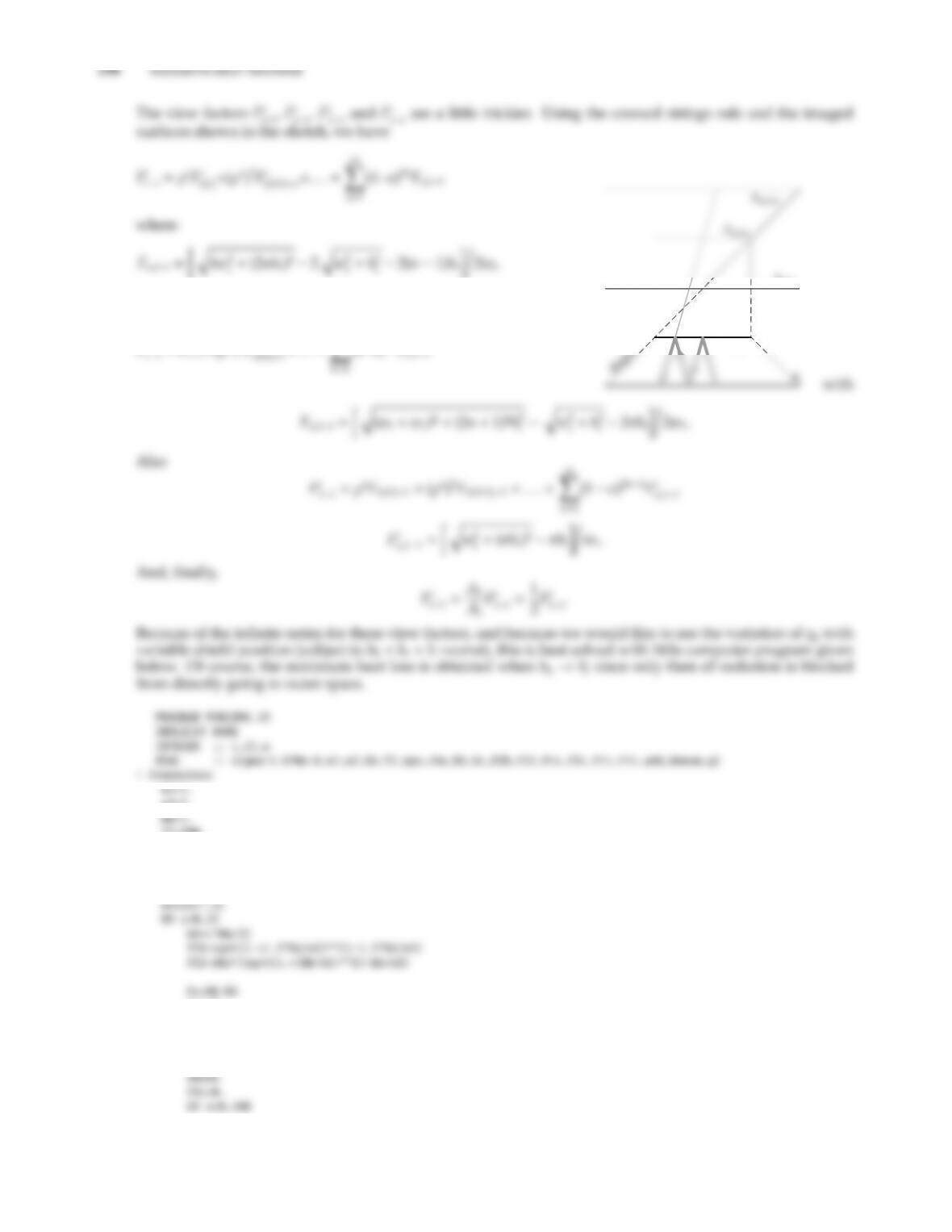Similarly,
Fs
X
(1ǫ)2nFn,1t
A1
At(1)
side
w2=2.
T2=600.
eps=.1
rho=1.-eps
II=20
! Loop over different hb
Ftt=0.
DO n=1,1000.05 695.18
0.15 1019.81
0.25 1326.99
0.35 1616.97
0.45 1890.11
0.55 2146.83
0.65 2387.36
0.75 2611.72
0.85 2818.67
0.95 3002.27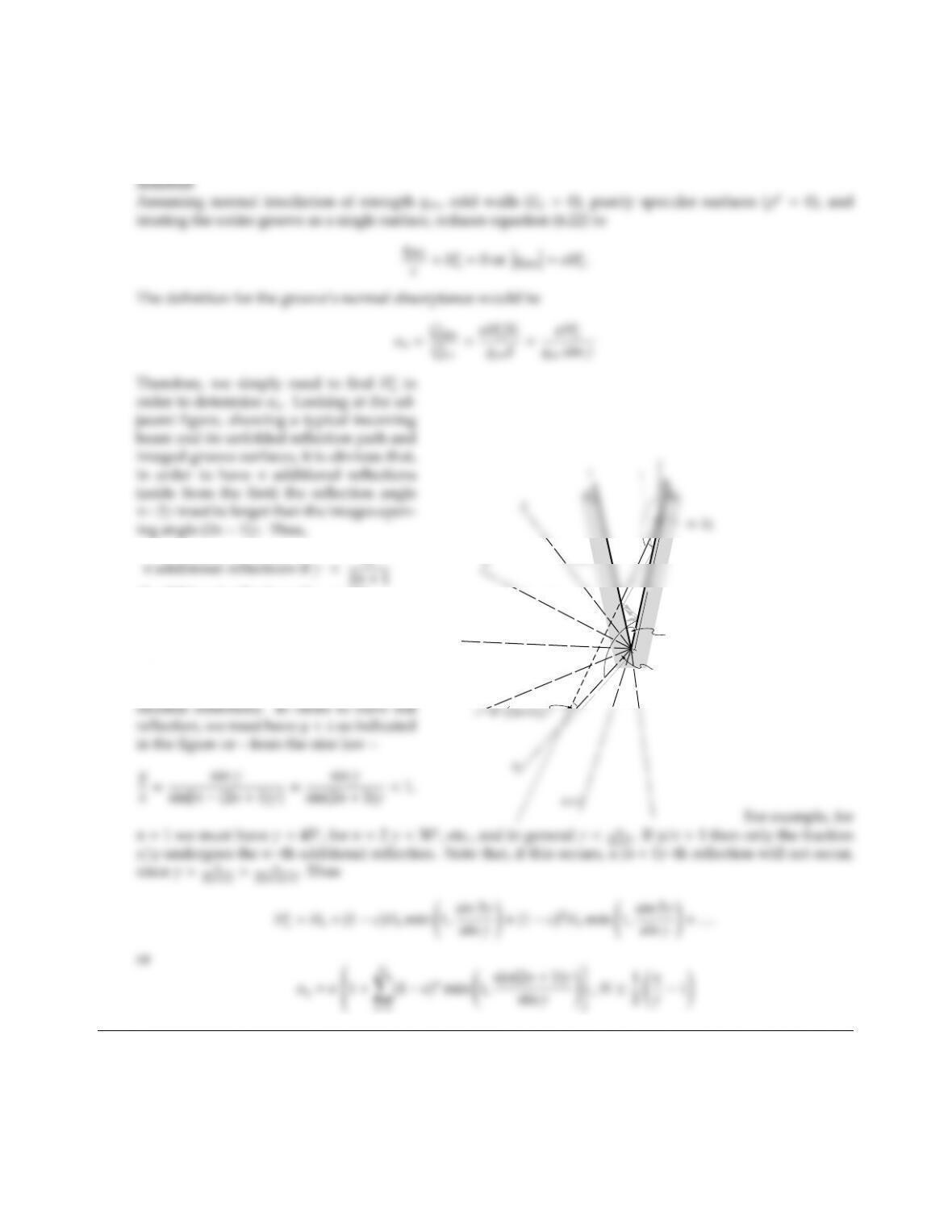6.22 Evaluate the normal emittance for the V-corrugated surface shown in Fig. 6-10a. Hint: This is most easily
calculated by determining the normal absorptance, or the net heat ﬂux on a cold groove irradiated by parallel
light from the normal direction.
(1 additional reﬂections if γ < 60,
2 additional reﬂections if γ < 30,etc.).
If an nth additional reﬂection occurs, it
may be partial or full (i.e. all incoming
beams hitting “0” undergo the nth ad-
A1(2)
A2(12)
A1(212) 2
γ
4
y
x
γ
γ
2n6.23 Redo 6.22 for an arbitrary o-normal direction 0 < θ < π/2 in a two-dimensional sense (i.e., what is the
o-normal absorptance for parallel incoming light whose propagation vector is in the same plane as all the
surface normal, namely the plane of the paper in Fig. 6-10).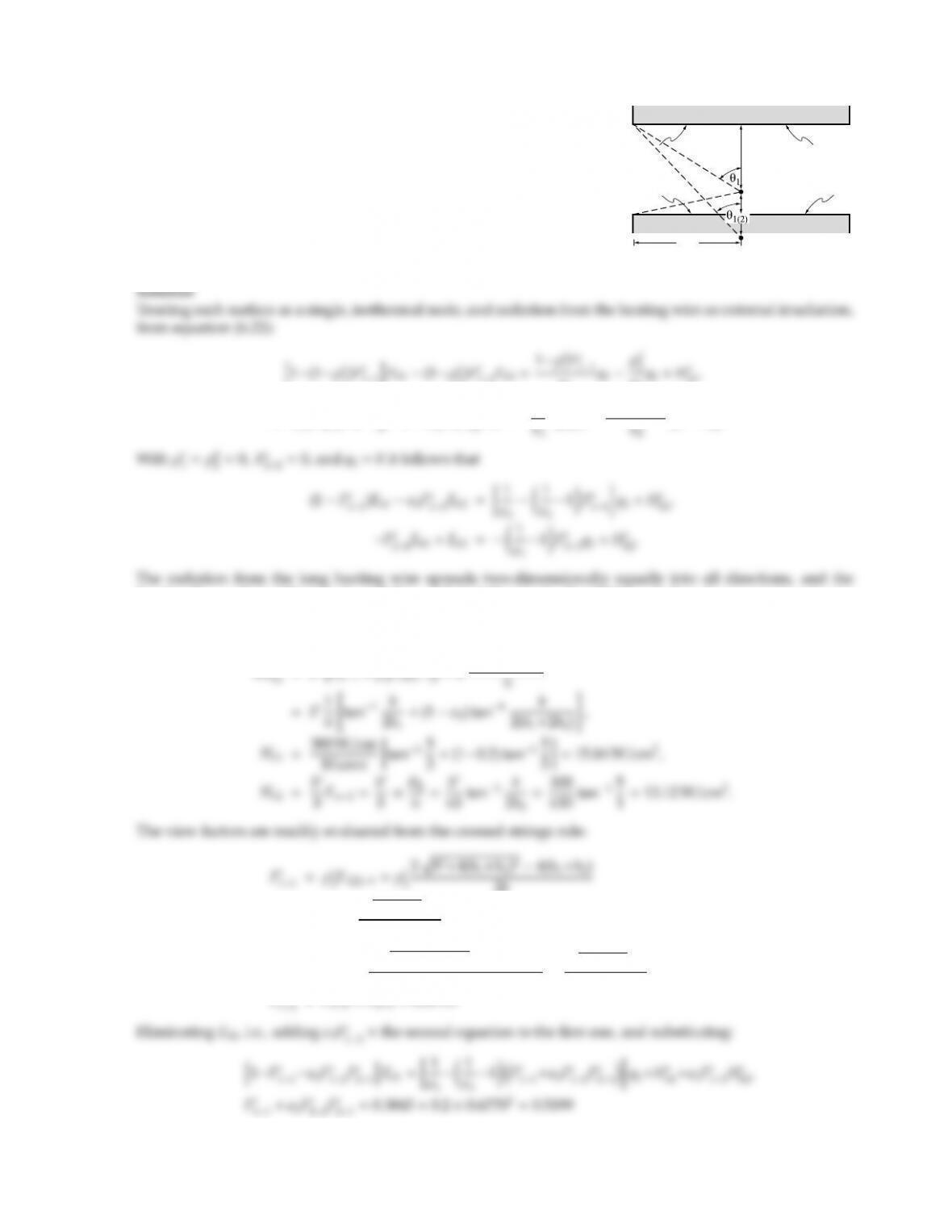6.24
A2
T2, 2
q1, 1
b/2
A1
h2 = 1cm
S'
S'(2)
A long, thin heating wire, radiating energy in the amount of S=
300 W/cm (per cm length of wire), is located between two long, parallel
plates as shown in the adjacent sketch. The bottom plate is insulated
and specularly reﬂecting with ǫ2=1ρs
2=0.2, while the top plate is
isothermal at T1=300 K and diusely reﬂecting with ǫ1=1ρd
1=0.5.
Determine the net radiative heat ﬂux on the top plate.
1)Fs
11iEb1(1ρs
2)Fs
12Eb2=1ρd
ǫ1
ǫ2
o1,
(1ρs
1)Fs
21Eb1+h1(1ρs
2)Fs
22iEb2=ρd
1
Fs
21q1+1ρd
2Fs
22
q2+Hs
o2.
fraction Fw1=θ1falls into A1, and Fw2=θ2falls into A2. In addition, surface A1sees a mirror image
of the wire behind A2. Thus, A1gets additional, external irradiation of the amount ρs
2Fw(2)1=ρs
2θ1(2). The
external contributions are then
bHs
2θ1(2)
2b
=0.8×100+648
10 =0.3845,
Fs
21=F21=2pb2+(h1+h2)22(h1+h2)
2b=100+164
10 =0.6770,
Fs

## Trusted by Thousands ofStudents

Here are what students say about us.